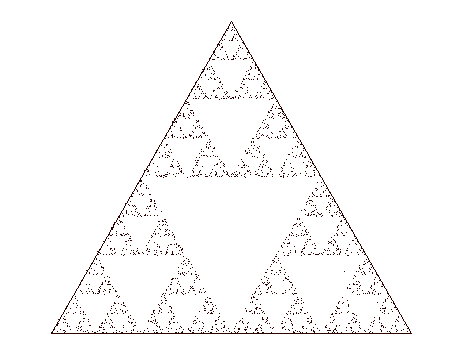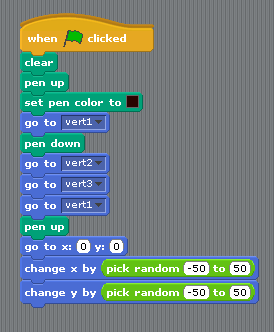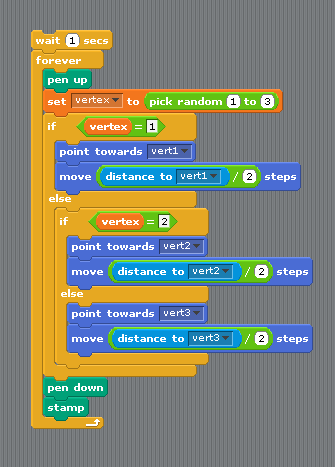# Scratch ProgrammingSierpinksi Triangle

## Introduction

The Sierpinksi Triangle is a special and interesting shape. Read all about it at Wikipedia - http://en.wikipedia.org/wiki/Sierpinski_triangle

This program uses the Chaos Game method to draw the triangle. It does the drawing dot-by-dot and you end up with something like this,## The Code

This is quite a long script so it has been chopped in two for this page. The first section draws an equilateral triangle. Each vertex or point in the triangle has a 1pixel square sprite. Code has been added to the sprites to arrange them in an equilateral triangle. Download the program and you will see how that was done.This script does the drawing.